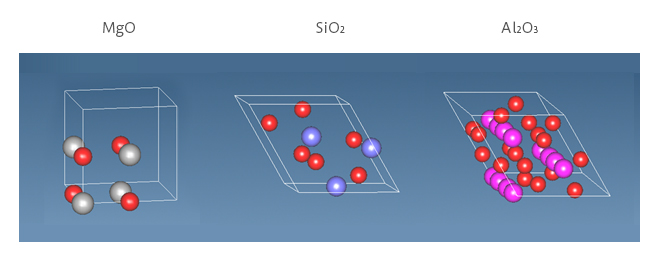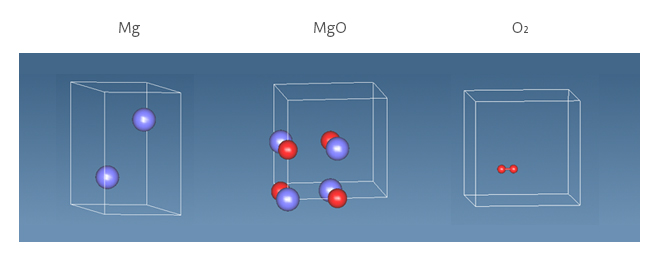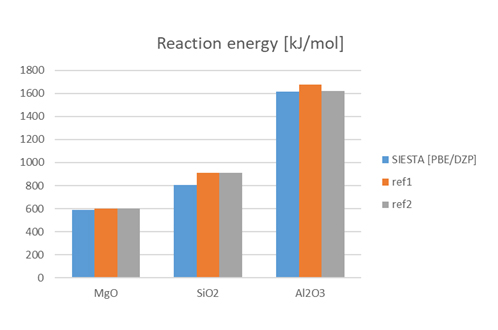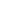Quantum Chemistry / DFT
Other Properties
Materials Science

# Formation energy of reaction

## Purpose and method

The oxidation reaction energies (ΔE) of the three metals shown in Figure 1 were calculated.

Mg + 1/2 O2 → MgO

Si + O2 → SiO2

2Al + 3/2 O2 → Al2O3

The reaction energy (ΔE) is calculated from the following formula and corresponds to the formation energy of oxide. The calculation models for oxidation of Mg are shown in Figure 2. ΔE is obtained from the difference between the energy of the reactants and the energy of the products. The calculation was performed using SIESTA, and PBE was applied to the functional and DZP (EnergyShift = 0.2 eV) was applied to the basis function. (MeshCutoff: 300.0 Ry, kgrid_cutoff: 10.0 angstrom)

E(Mg) + 1/2 E(O2) = E(MgO) + ΔE

E(Si) + E(O2) = E(SiO2) + ΔE

E(2Al) + 3/2 E(O2) = E(Al2O3) + ΔEFigure 1. Simulation model of oxideFigure 2. Simulation models for reaction energy of MgO

## Simulation result

The results are shown in Figure 3. All of results were in good agreement with the reference values. This indicates that SIESTA can evaluate the energy change during the reaction.
* The reference value is the enthalpy of oxide formation (ΔH = ΔE + ΔPV) and not exactly the same meaning as the calculated value, but it is compared as an approximate value because the contribution of PV is considered to be small in the case of solids.Figure 3. Comparison of calculation results of reaction energy by SIESTA with references
ref1: https://doi.org/10.1155/2014/120840
ref2: NIST Chemistry Webbook (https://doi.org/10.18434/T4D303)This website uses cookies to improve functionality and performance. If you continue browsing the site, you are giving implied consent to the use of cookies on this website. If you want to know more or refuse consent, read our Cookie Policy.

Accept